# AP Chemistry : Atomic Structure and Properties

## Example Questions

### Example Question #31 : Elemental Composition Of Pure Substances

What is the formula of hydroiodic acid?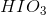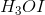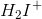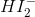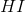Explanation:is the correct formula because, following IUPAC acid naming rules, when combining a hydrogen cation with an anion that consists of only one element (in this case iodide), one drops the -ide ending, adds an -ic ending and adds a hydro- prefix. Other examples would be hydrochloric acid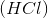and hydrofluoric acid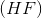.

### Example Question #32 : Elemental Composition Of Pure Substances

A student named a certain organic molecule 2-(1-methylbutyl)-3-isopropylbutane. While this name may give the possibility of correctly drawing the molecule, it does not follow correct IUPAC naming guidelines. What is the correct name of this molecule?

3,4-dimethyl-2-isopropylheptane

3-(2-pentyl)-2-isopropylbutane

2-(2-pentyl)-3-isopropylbutane

2,3,4,5-tetramethyloctane

2-pentyl-3-propylbutane

2,3,4,5-tetramethyloctane

Explanation:

While the student's description of the location and size of the sidechains is correct, he/she failed to recognize the longest parent chain, it being the eight-carbon string which encompasses what he/she named isopropyl- and pentyl-. The resulting octane molecule has four methyl- substituents at the 2, 3, 4, and 5 positions.

### Example Question #33 : Elemental Composition Of Pure Substances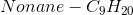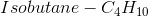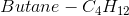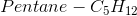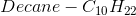Which of the molecules above does not match its molecular formula?

Butane

Isobutane

Pentane

Decane

Nonane

Butane

Explanation:

While it is tempting to think that butane and isobutane, because of their structural differences would have different numbers of of hydrogens, they are in fact constitutional isomers, and have the same molecular formula. Both butane and isobutane have four carbons and ten hydrogens, although butane's carbons and hydrogen's are arranged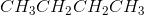and isobutane's are arranged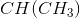A good rule of thumb for the formulas of simple hydrocarbons is: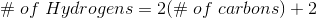### Example Question #34 : Elemental Composition Of Pure Substances

Which of the following is a correct name for the compound,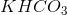?

I: Potassium bicarbonate

II: Potassium percabonate

III: Potassium hydrogen carbonate

I only

III only

II only

I and III

II and III

I and III

Explanation:

Because the ion,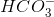, is correctly referred to as both bicarbonate and hydrogen carbonate, the correct names for the compound in question are both potassium bicarbonate and potassium hydrogen carbonate.

### Example Question #35 : Elemental Composition Of Pure Substances

Which of these is not the IUPAC name for the indicated molecule?

Perchlorous acid;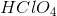Hydrofluoric acid;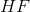Sulfuric acid;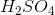Carbonous acid;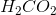Acetic acid;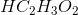Perchlorous acid;Explanation:

Because the polyatomic ion from which the acid,, is derived is perchlorate,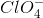, the resulting acid is named perchloric acid. The naming protocol is to use the -ic suffix for acids derived from polyatomic ions ending in -ate, while using the -ous suffix for acids derived from polyatomic ions ending in -ite.

### Example Question #91 : Atomic Structure And Properties

What is the name of the compound with the chemical formula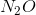?

Pernitrate

Nitrite

Nitrate

Hyponitrite

Dinitrogen monoxide

Dinitrogen monoxide

Explanation:

All of the incorrect options have prefixes or suffixes associated with polyatomic ions "hypo," "per," "ite," and "ate". Sinceis not an ion (it does not have a charge), it must just be a covalent compound. Therefore, use the prefixes reserved for covalent compounds to name it.

### Example Question #37 : Elemental Composition Of Pure Substances

What is the name of the compound with the chemical formula: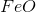?

Iron (I) oxide

Iron monoxide

Iron oxide

Iron (II) oxide

Iron (III) oxide

Iron (II) oxide

Explanation:

The name of this compound is iron (II) oxide. First, notice how the chemical formulacontains one metal and one nonmetal, so we know that we are not going to be using prefixes such as "mono" because those are used for covalent compounds. Secondly, notice that iron is a transition metal, which means that we have to specify the charge it has, in roman numerals. Iron must have a positive two charge to balance out the negative two charge oxygen will have.

### Example Question #38 : Elemental Composition Of Pure Substances

Name of the following compound: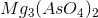Magnesium perarsenate

Magnesium(II) perarsenate

Magnesium(II) aresenate

Magnesium arsenite

Magnesium arsenate

Magnesium arsenate

Explanation:

This is an ionic compound, we know that because we see a metal bound to a nonmetal. To name ionic compounds, first write the name of the metal, or cation: magnesium. Next, we have the polyatomic ion arsenate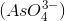. Thus our answer is: magnesium arsenate. Since magnesium is not a transition metal, we do not need to indicate its charge with Roman numerals. If arsenate was part of a series of oxyanions (anions that contain oxygen), we use the prefix "per" to indicate more oxygens, however this is not the case.

### Example Question #39 : Elemental Composition Of Pure Substances

What is the name of the following compound?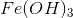Iron trihydroxide

Iron (III) hydroxide

Ferrous hydroxide

Iron (II) hydroxide

Iron (III) hydroxide

Explanation:

To name this compound, it's important to first notice what the formal charge would be on each of the constituent ions. We know that the formal charge of a hydroxide ion is. Since there are three of these, and because we know the compound is neutral, the iron constituent must have a charge of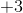. Therefore, this compound is correctly called iron (III) hydroxide.

It's also important to note that there are alternate names for iron (II) and iron (III). Iron (II) is sometimes referred to as "ferrous" while iron (III) is sometimes referred to as "ferric." An easy way to remember this is to note that "ferrous" has an "O" in it and so does the word "lower" (lower as in the lower charge of the +2 iron). Moreover, the term "ferric" has an "I" in it and so does the word "higher" (higher as in the higher charge of +3 iron).

### Example Question #40 : Elemental Composition Of Pure Substances

Which of the following give the correct name for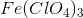?

Iron(III) Perchlorate

Iron(III) Perchlorite

Iron(II) Perchlorate

Iron(III) Chlorate

Iron(III) Perchlorate

Explanation:

This question is asking us to identify the correct name for a given compound.

To begin, we first need to identify what cation and anion are in the compound given,. For ionic compounds, the cation(s) is/are written first, followed by the anion(s).

Let's look at the anion first. We can see that there are three equivalents of anions in this compound because there are a total of three. When deciding on the name of the anion, we can see that it is a halogen bound to four oxygens. Because it is bound to four oxygens, it is called perchlorate. If it were bound to three oxygens, it would be called chlorate. If it were two it would be called chlorite, and if it were only one it would be called hypochlorite.

In addition, since the anion has a charge of, and because there are three of them for every one cation, then the cation must have a charge ofin order for the compound to be neutral. Thus, the cation in this case will be iron(III).

Putting these two together, we arrive at iron(III) perchlorate as the correct name for this compound.